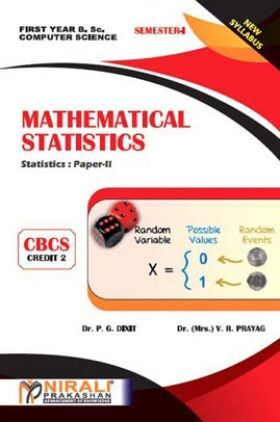# Mathematical Statistics

Sold ( 51 times )
1375 Views
MRP : ₹100.00
Price : ₹66.50
You will save : ₹33.50 after 34% Discount
Inclusive of all taxes

Save extra with 2 Offers

Get ₹ 50

Instant Cashback on the purchase of ₹ 400 or above

Product Specifications

 Publisher Nirali Prakashan All B.Sc. Mathematics books by Nirali Prakashan ISBN 9789389108583 Author: Dr. (Mrs.) V. R. Prayag, Dr. (Mrs.) V. R. Prayag Number of Pages 201 Edition First Edition Available Available in all digital devicesMathematical Statistics by Dr. (Mrs.) V. R. Prayag, Dr. (Mrs.) V. R. Prayag
Book Summary:

We feel indeed very happy to present this text-book of 'Statistics Paper-II' Mathematical Statistics to the students of F.Y.B.Sc. Computer Science. The book is written according to the revised syllabus with effect from June, 2019.

The main purpose of the book is to provide foundation as well as comprehensive background of Probability Theory and statistical methods to beginners in simple and interesting manner. In order to make the contents of the book easier to comprehend, we have included a requisite number of illustrations, remarks, figures, diagrams etc. to elucidate statistical concepts. Application of Statistics in real life situations is emphasized through illustrative examples. Ample number of graded problems, theoretical as well as numerical are provided at the end of each chapter along with hints and answers. The numerical problems will also be useful for the F.Y.B.Sc. computer science students to prepare for Paper – III : Practicals. A list of practicals is given in the syllabus. Appendix A compiles some important mathematical results which are needed during the entire course. Values of individual terms of binomial probabilities are given in Appendix B. A specimen paper is set for student's self assessment.

Audience of the Book :
This book Useful for B.Sc Computer Science Students.
Table of Content:

1. Revision of Theory of Probability

2. Conditional Probability & Baye's Theorem

3. Random Variables

4. Mathematical Expectation

5. Standard Discrete Distribution

Model Question Paper